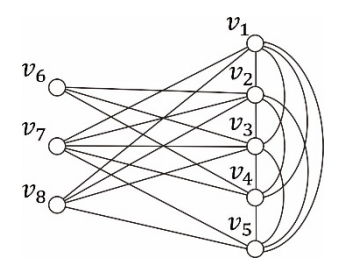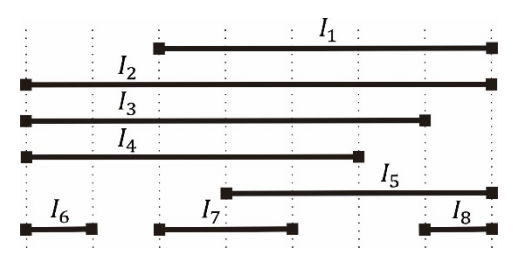시간 제한메모리 제한제출정답맞힌 사람정답 비율
3 초 512 MB88311728.333%

## 문제

Network vulnerability refers to how much the network performance reduces in various cases of disruptions, such as natural disasters, element failures, or adversarial attacks. A network is frequently represented as a graph, in which the vertices and edges correspond to nodes and links, respectively. So the terms network and graph are used interchangeably here. The connectivity, which is defined to be the minimum number of vertices whose deletion results in a disconnected graph or a one-vertex graph, is recognized as the most important measure to evaluate the vulnerability of a network.

Nonetheless, the connectivity only partly reflects the resistance of a graph to the deletion of vertices. Accordingly, there have been many studies proposing other metrics to account for the network vulnerability, among which the toughness and the scattering number appear to be the most popular. The underlying notion of the toughness and scattering number is the maximum number of connected components resulting from removing k vertices from a graph. Let ck(G) denote the maximum number of connected components obtained by removing exactly k vertices from a graph G. It is unfortunate that given a graph G and a positive integer k, the problem of determining ck(G) is computationally intractable.

For some graph classes like interval graphs, however, ck(G) can be computed in polynomial time. An interval graph is the intersection graph of a family of (closed) intervals on the real line, where two vertices are connected with an edge if and only if their corresponding intervals intersect. The family is usually called an interval representation for the graph. See Figure E.1 for an example of an interval graph and its interval representation.(a) an interval graph (b) an interval representation of the graph of (a)

Given an interval representation of an interval graph G with n vertices, your job is to write an efficient running program for computing ck(G) for all k included in {0, 1, … , k − 1}. If the interval representation shown in Figure E.1(b), for example, is given, then ܿc0(G), c1(G), c2(G), c3(G), c4(G), c5(G), c6(G), c7(G) are 1, 1, 1, 2, 2, 3, 2, 1, respectively.

## 입력

Your program is to read from standard input. The first line contains a positive integer n representing the number of intervals, where n ≤ 2,000. In the following n lines, each contains a pair of left and right endpoints of an interval. You may assume that the endpoints, left or right, of intervals are integers between −100,000,000 and 100,000,000, inclusive.

## 출력

Your program is to write to standard output. Print exactly one line which contains the sequence ܿc0(G), c1(G), ..., cn-1(G) of n numbers separated by a single space.

## 예제 입력 1

8
3 8
1 8
1 7
1 6
4 8
1 2
3 5
7 8


## 예제 출력 1

1 1 1 2 2 3 2 1


## 예제 입력 2

3
-2 -2
-2 7
-2 7


## 예제 출력 2

1 1 1


## 예제 입력 3

4
-1 -1
3 4
5 6
7 8


## 예제 출력 3

4 3 2 1


## 예제 입력 4

5
1 2
2 3
3 4
6 7
7 8


## 예제 출력 4

2 3 3 2 1